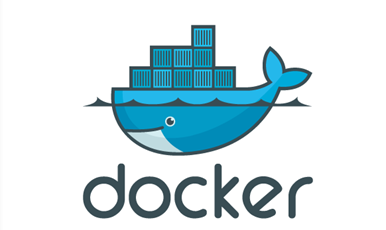# .Net Core 实现图片验证码的实现示例_实用技巧

Net Standard库是.Net Core可移植性的核心。Net Standard是标准。.Nprivate static string RndNum(int VcodeNum) { //验证码可以显示的字符集合 string Vchar = "0,1,2,3,4,5,6,7,8,9,a,b,c,d,e,f,g,h,i,j,k,l,m,n,p" + ",q,r,s,t,u,v,w,x,y,z,A,B,C,D,E,F,G,H,I,J,K,L,M,N,P,P,Q" + ",R,S,T,U,V,W,X,Y,Z"; string[] VcArray = Vchar.Split(new Char[] { ',' });//拆分成数组 string code = "";//产生的随机数 int temp = -1;//记录上次随机数值，尽量避避免生产几个一样的随机数 Random rand = new Random(); //采用一个简单的算法以保证生成随机数的不同 for (int i = 1; i < VcodeNum + 1; i++) { if (temp != -1) { rand = new Random(i * temp * unchecked((int)DateTime.Now.Ticks));//初始化随机类 } int t = rand.Next(61);//获取随机数 if (temp != -1 && temp == t) { return RndNum(VcodeNum);//如果获取的随机数重复，则递归调用 } temp = t;//把本次产生的随机数记录起来 code += VcArray[t];//随机数的位数加一 } return code; }public static MemoryStream Create(out string code, int numbers = 4) { code = RndNum(numbers); //Bitmap img = null; //Graphics g = null; MemoryStream ms = null; Random random = new Random(); //验证码颜色集合 Color[] c = { Color.Black, Color.Red, Color.DarkBlue, Color.Green, Color.Orange, Color.Brown, Color.DarkCyan, Color.Purple }; //验证码字体集合 string[] fonts = { "Verdana", "Microsoft Sans Serif", "Comic Sans MS", "Arial", "宋体" }; using (var img = new Bitmap((int)code.Length * 18, 32)) { using (var g = Graphics.FromImage(img)) { g.Clear(Color.White);//背景设为白色 //在随机位置画背景点 for (int i = 0; i < 100; i++) { int x = random.Next(img.Width); int y = random.Next(img.Height); g.DrawRectangle(new Pen(Color.LightGray, 0), x, y, 1, 1); } //验证码绘制在g中 for (int i = 0; i < code.Length; i++) { int cindex = random.Next(7);//随机颜色索引值 int findex = random.Next(5);//随机字体索引值 Font f = new Font(fonts[findex], 15, FontStyle.Bold);//字体 Brush b = new SolidBrush(c[cindex]);//颜色 int ii = 4; if ((i + 1) % 2 == 0)//控制验证码不在同一高度 { ii = 2; } g.DrawString(code.Substring(i, 1), f, b, 3 + (i * 12), ii);//绘制一个验证字符 } ms = new MemoryStream();//生成内存流对象 img.Save(ms, ImageFormat.Jpeg);//将此图像以Png图像文件的格式保存到流中 } } return ms; }function GetCode() { \$.ajax({ type: "GET", url: "/Users/Login/GetVerifyCode", data: {}, dataType: "json", success: function (data) { }, complete: function () { \$("#code").attr('src', '/Users/Login/GetVerifyCode') } }); }

SkiaSharp

public IActionResult Code() { #region 反射SK支持的全部颜色 //List<SKColor> colors = new List<SKColor>(); //var skcolors = new SKColors(); //var type = skcolors.GetType(); //foreach (FieldInfo field in type.GetFields()) //{ // colors.Add( (SKColor)field.GetValue(skcolors)); //} #endregion //int maxcolorindex = colors.Count-1; string text = "1A3V"; var zu = text.ToList(); SKBitmap bmp = new SKBitmap(80, 30); using (SKCanvas canvas = new SKCanvas(bmp)) { //背景色 canvas.DrawColor(SKColors.White); using (SKPaint sKPaint = new SKPaint()) { sKPaint.TextSize = 16;//字体大小 sKPaint.IsAntialias = true;//开启抗锯齿 sKPaint.Typeface = SKTypeface.FromFamilyName("微软雅黑", SKTypefaceStyle.Bold);//字体 SKRect size = new SKRect(); sKPaint.MeasureText(zu.ToString(), ref size);//计算文字宽度以及高度 float temp = (bmp.Width/4 - size.Size.Width)/2; float temp1 = bmp.Height - (bmp.Height - size.Size.Height) / 2; Random random = new Random(); for (int i = 0; i < 4; i++) { sKPaint.Color = new SKColor((byte)random.Next(0,255), (byte)random.Next(0, 255), (byte)random.Next(0, 255)); canvas.DrawText(zu[i].ToString(), temp + 20*i, temp1, sKPaint);//画文字 } //干扰线 for (int i = 0; i < 5; i++) { sKPaint.Color = new SKColor((byte)random.Next(0, 255), (byte)random.Next(0, 255), (byte)random.Next(0, 255)); canvas.DrawLine(random.Next(0, 40), random.Next(1, 29), random.Next(41, 80), random.Next(1, 29), sKPaint); } } //页面展示图片 using (SKImage img = SKImage.FromBitmap(bmp)) { using (SKData p = img.Encode()) { return File(p.ToArray(), "image/Png"); } } } }

netcore怎么实现三层架构

netstandard和.netcore的区别

• Net Standard库是.Net Core可移植性的核心。

• Net Standard是标准。.Net Core是这个标准的一种实现(此外，还有.Net Framework，Mono等实现方式)。

NET Core：

• 是免费、跨平台的，是托管框架的开源实现。

• 它支持4种类型的应用程序：控制台、ASP.NET Core，云和通用Windows平台（UWP）。Windows Forms和Windows Presentation Foundation（WPF）并不包含在.NET Core中。

• 从技术上讲，.NET Core仅支持控制台应用程序。ASP.NET Core和UWP是以.NET Core为基础构建的应用程序模型。

• NET Standard:

• 托管框架的每一种实现都有一套自己的基类库。基类库（BCL）包含诸如异常处理、字符串、XML、I/O、网络和集合这样的类。

• .NET Standard是一项实现BCL的规范。由于.NET实现需要遵循这项规范，所以应用程序开发人员就不用担心每一种托管框架实现的BCL不同。

• 框架类库（FCL），如WPF, WCF,ASP.NET，不包含在BCL中，因此，也就不包含在.NET Standard中。

• .NET Standard与.NET实现之间的关系就和HTML规范与浏览器之间的关系一样。后者是前者的实现。

netcore什么时候开始支持窗体程序

.Net Standard库是.Net Core可移植性的核心，其目的是定义标准的API集。 这些API，如集合、线程、反射，是由.Net Core、.Net 4.5、Xamarin和Mono等平台实现的，它们是开发人员用来构建自己的库和应用程序的基本构建块。本回答被提问者采纳

• 本文相关：
• .net core 2.0如何生成图片验证码完整实例
• asp.net+jquery+.ashx文件实现分页思路
• winform控件savefiledialog用于保存文件
• 状态保存机制之viewstate概述及应用
• 一个完整的asp.net 2.0 url重写方案[翻译]
• asp.net下xml当作导航数据源实现动态权限
• asp.net 分页控件源码
• iis中asp.net连接sql server出错的解决方法
• .net core应用类型（portable apps & self-contained apps）
• aspose.cells 读取受保护有密码的excel文件
• asp.net mvc5网站开发之实现数据存储层功能（三）
• netcore怎么实现三层架构
• netstandard和.netcore的区别
• netcore什么时候开始支持窗体程序
• 终于知道什么情况下需要实现.NET Core中的IOptions接口
• 什么功能或框架 在NETcore中能实现但JAVA实现不了
• netstandard和.netcore有哪些区别？
• 免责声明 - 关于我们 - 联系我们 - 广告联系 - 友情链接 - 帮助中心 - 频道导航